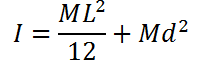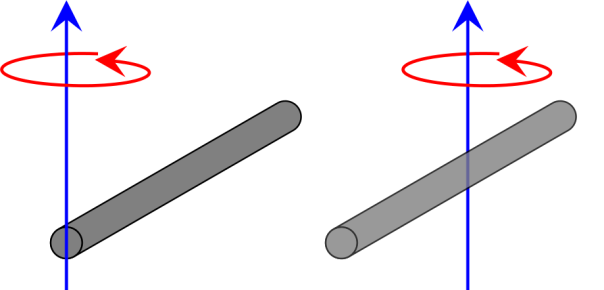# Moment of Inertia for Uniform Rod Calculation

This CalcTown calculator calculates the moment of inertia of a rod perpendicular to its longitudinal axis, at any distance form its centre of mass.

*Please enter 0 if the moment of Inertia is to be calculates about the centre of mass.

kg
m
m

#### Result

kg-m2